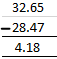# Selina Solutions Concise Maths Class 7 Chapter 4: Decimal Fractions (Decimals) Exercise 4B

## Selina Solutions Concise Maths Class 7 Chapter 4: Decimal Fractions (Decimals) Exercise 4B Download PDF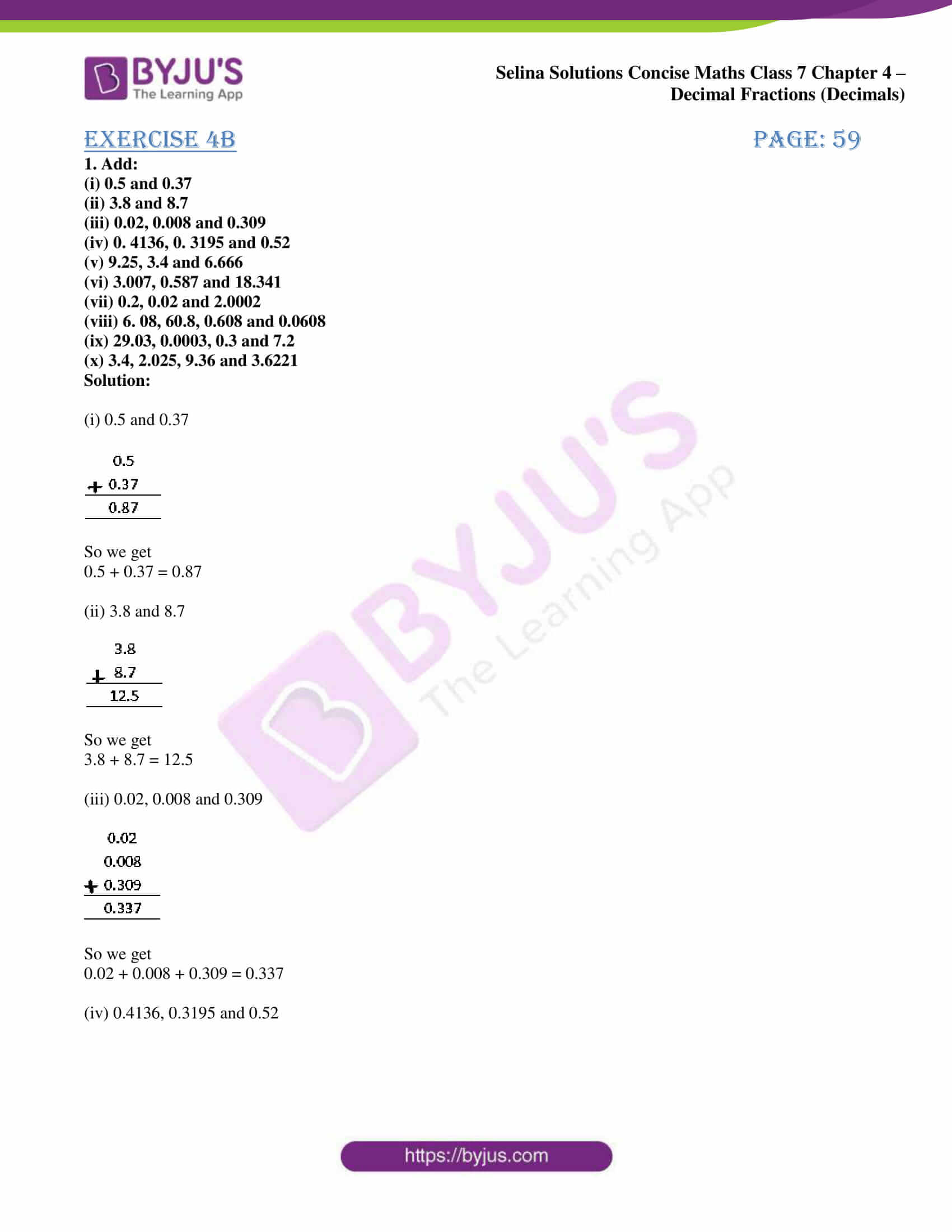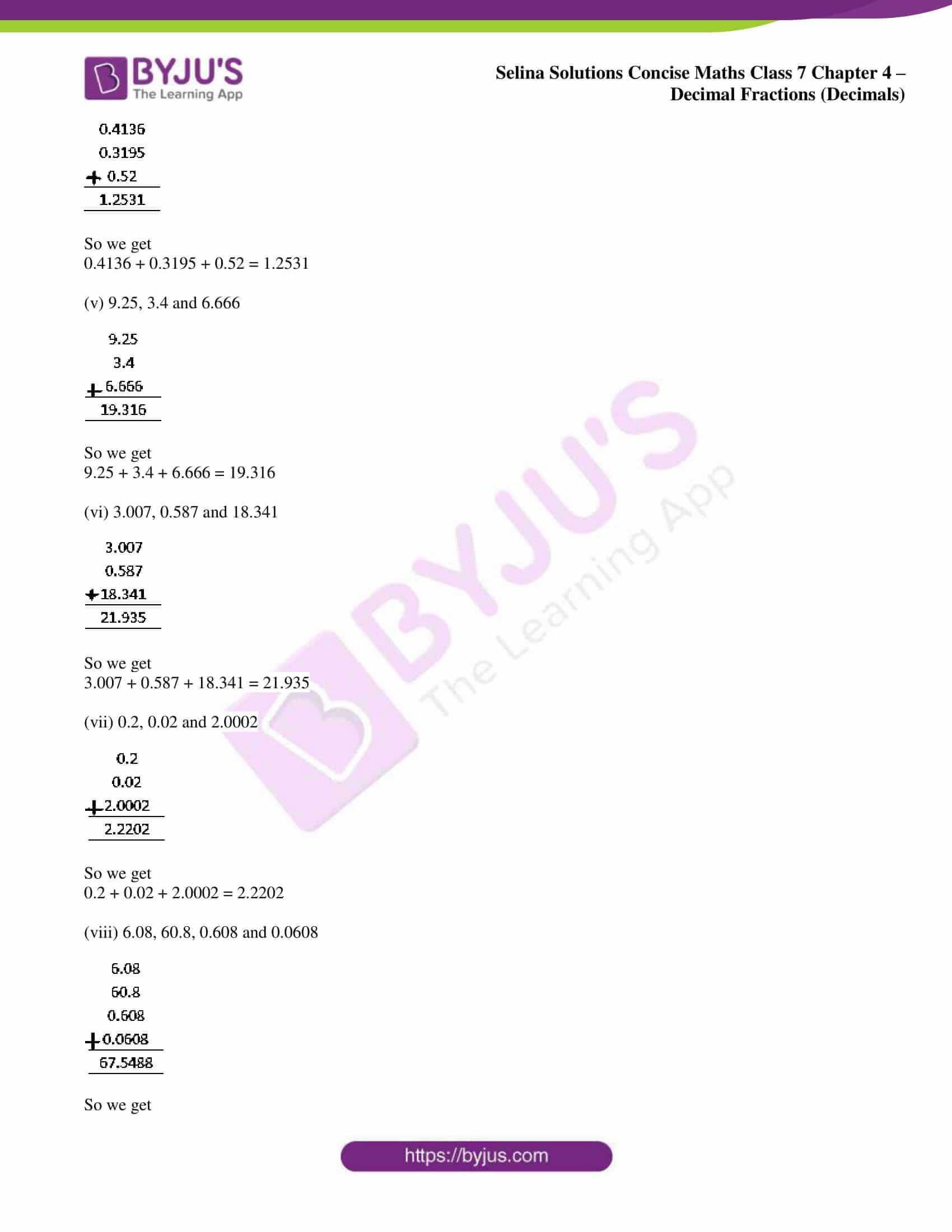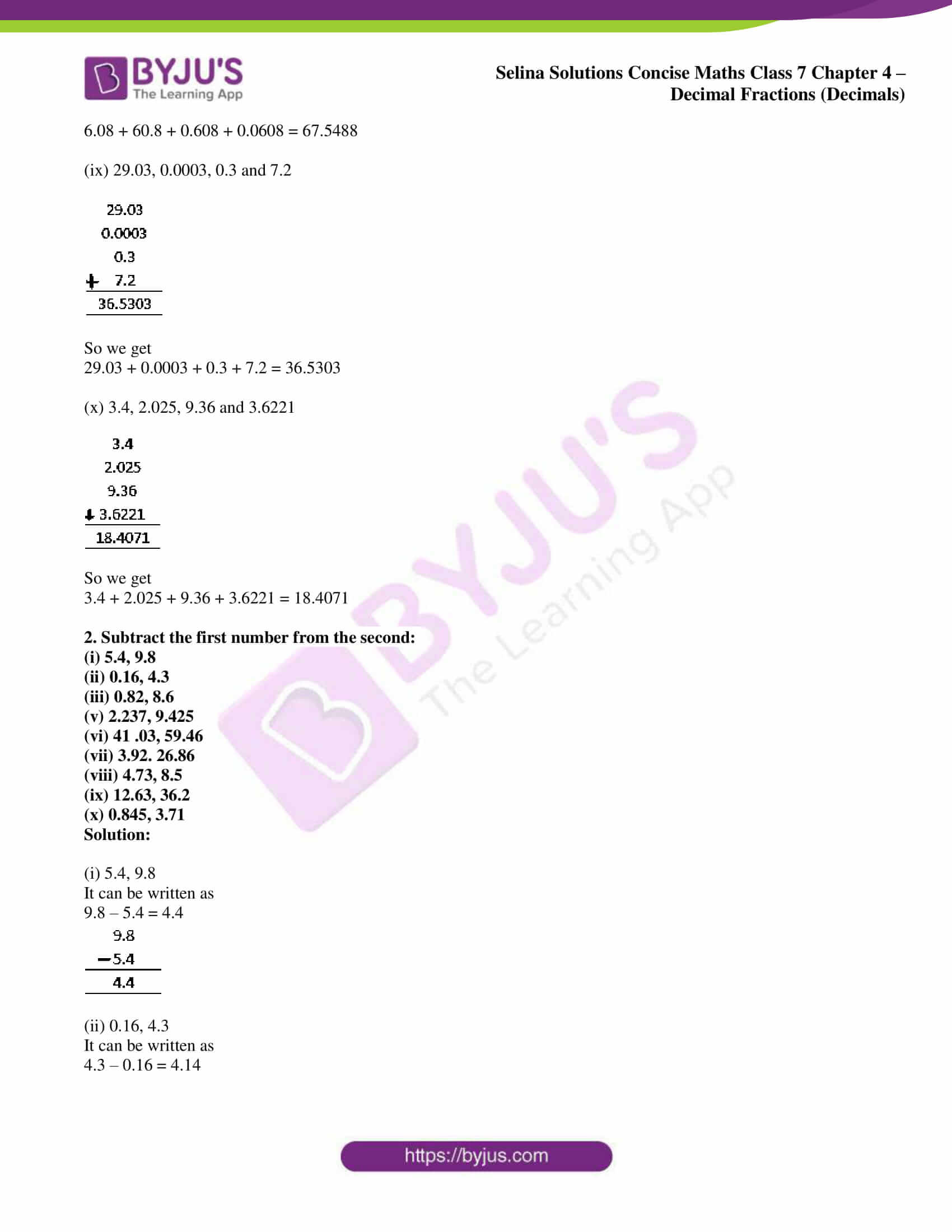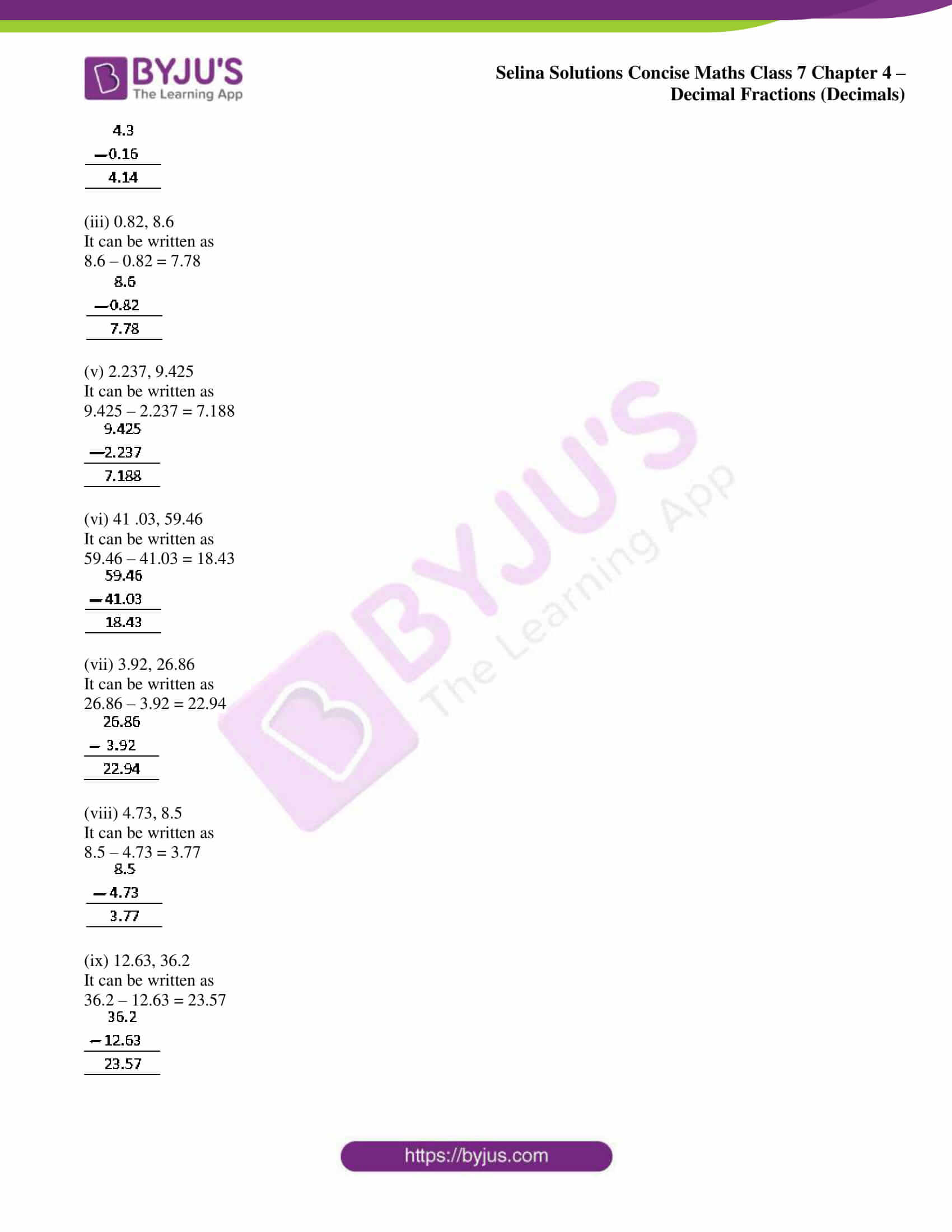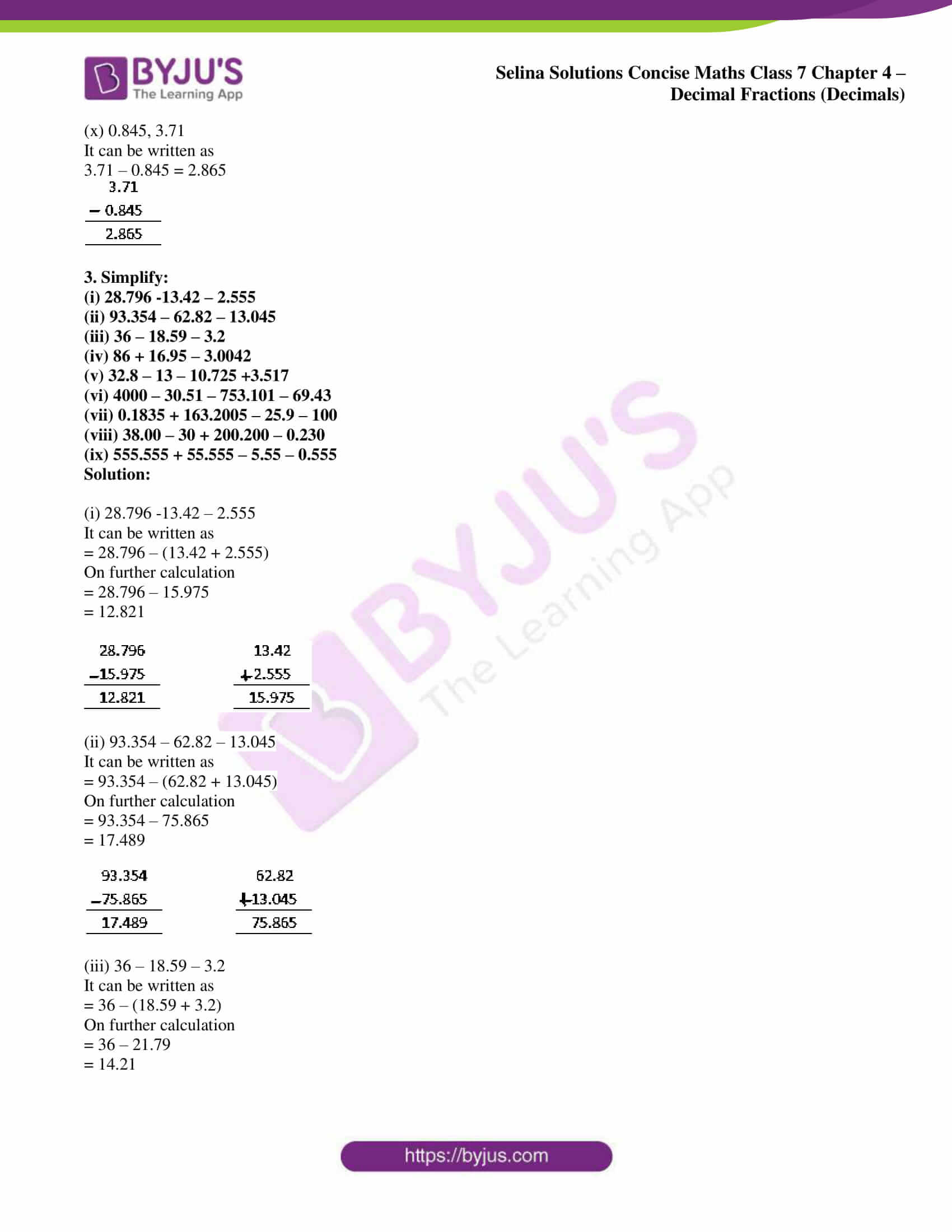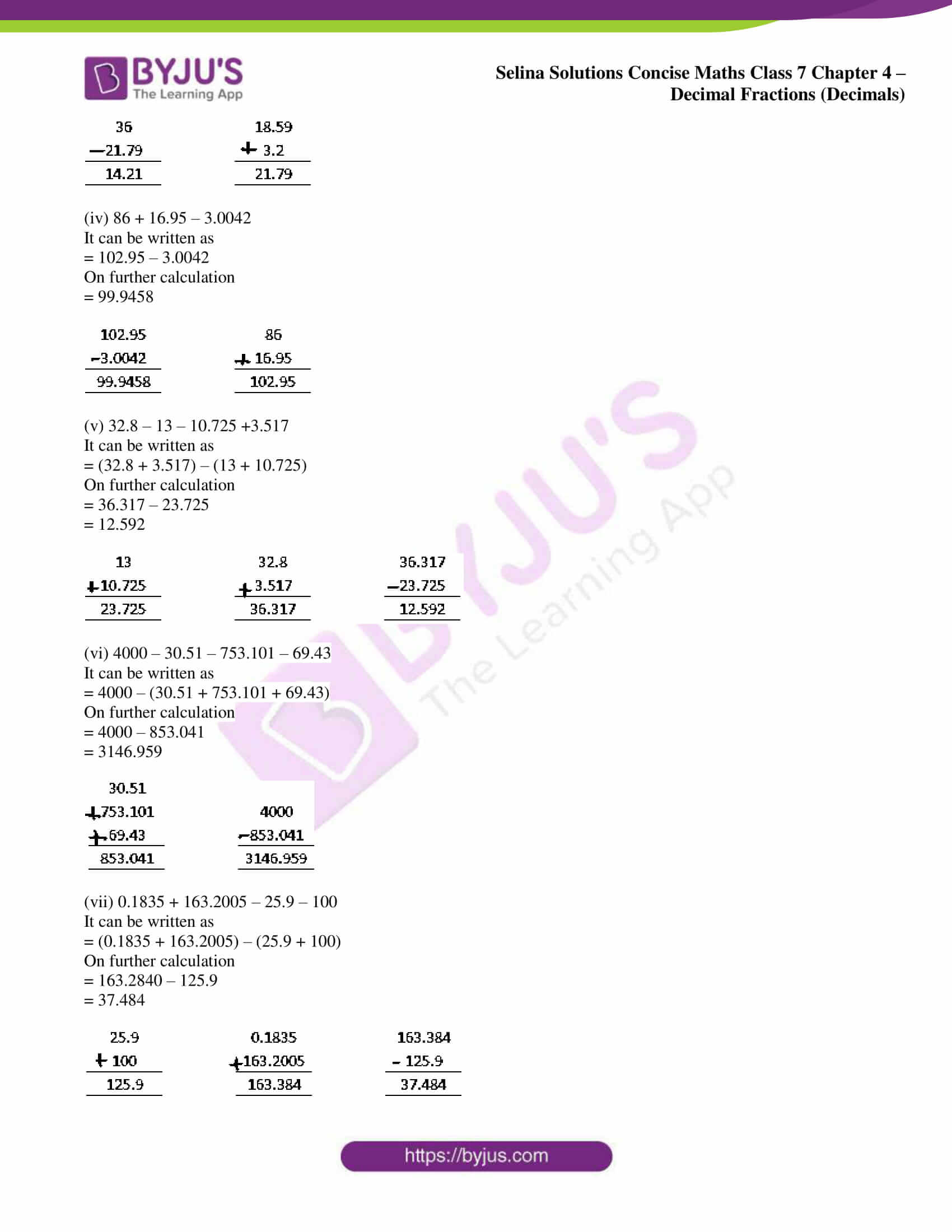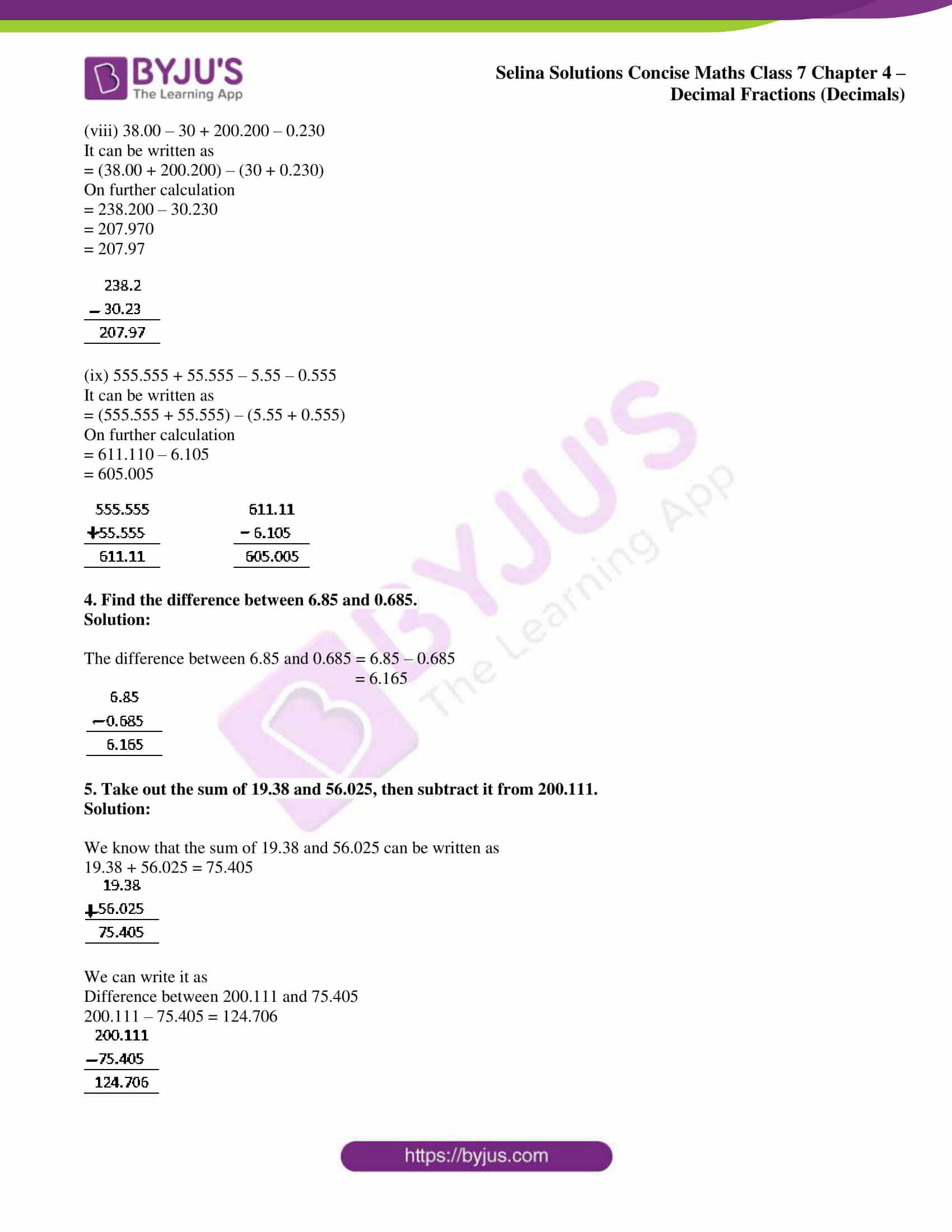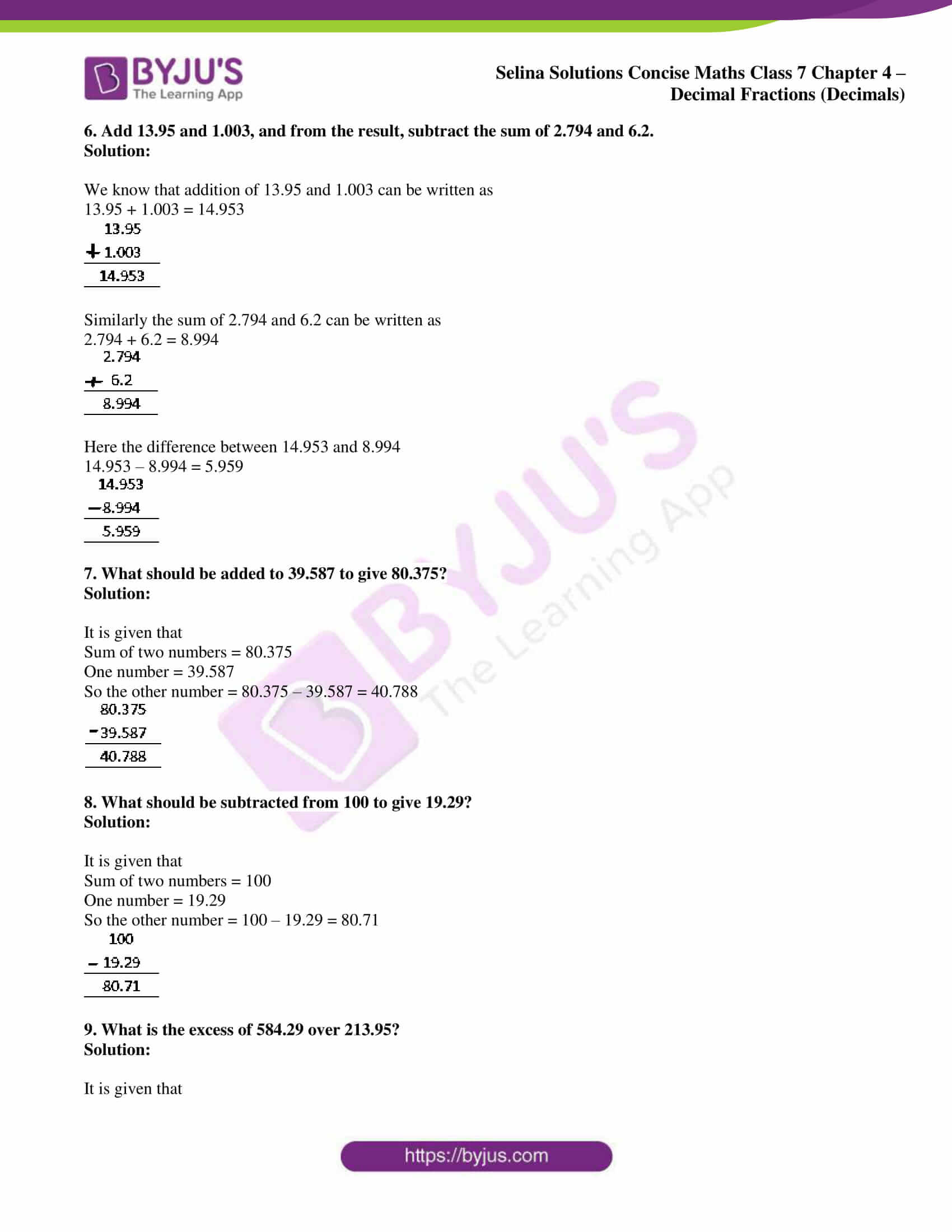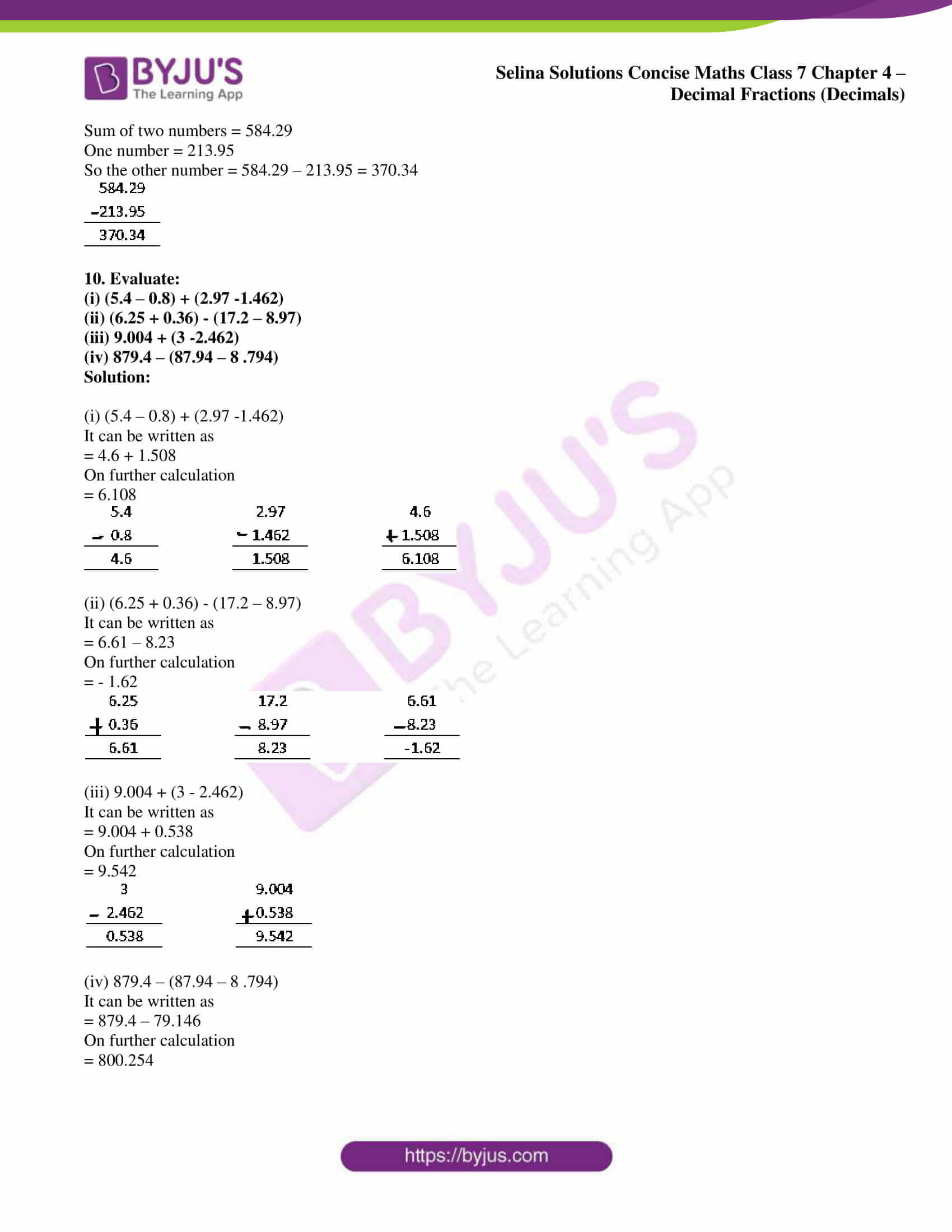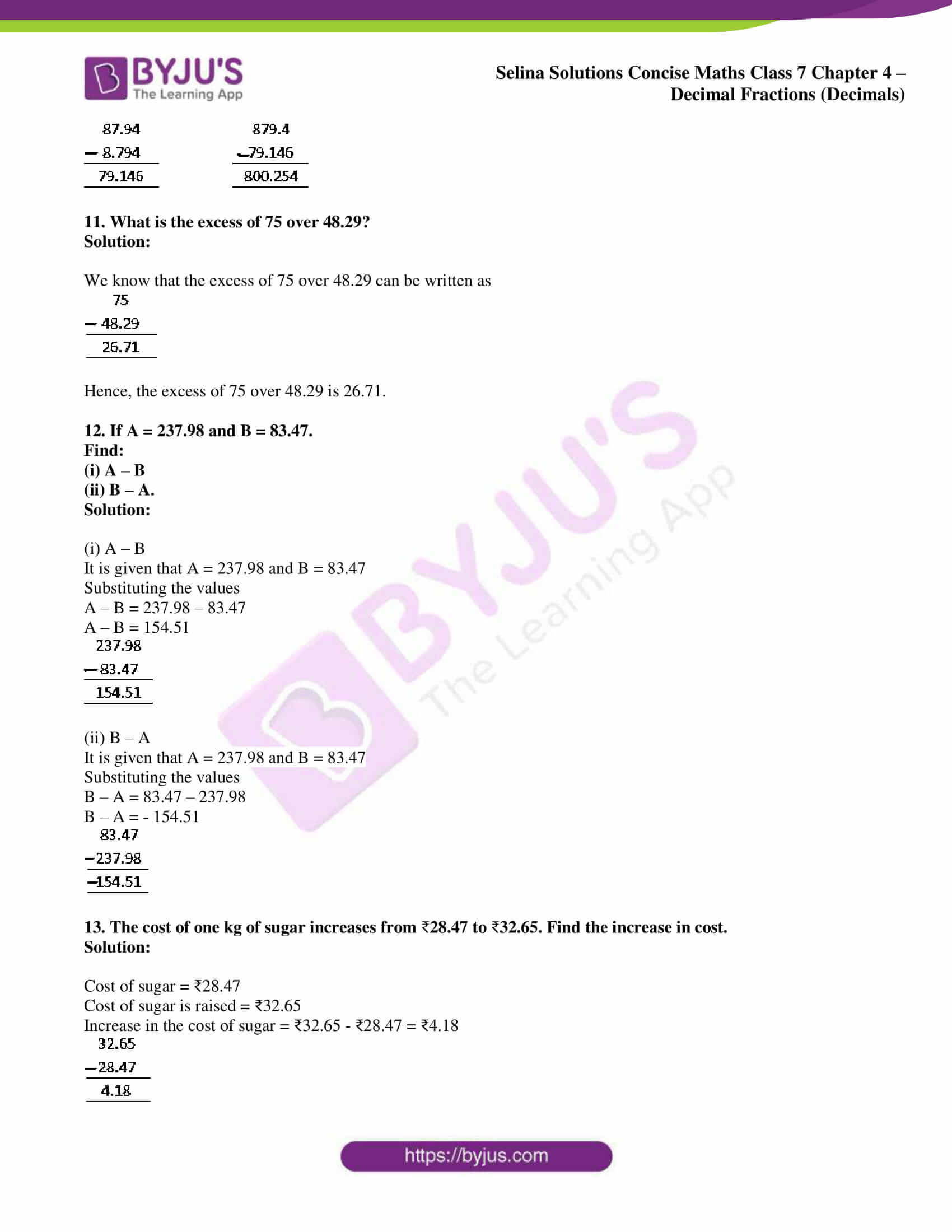### Access Selina Solutions Concise Maths Class 7 Chapter 4: Decimal Fractions (Decimals) Exercise 4B

(i) 0.5 and 0.37

(ii) 3.8 and 8.7

(iii) 0.02, 0.008 and 0.309

(iv) 0. 4136, 0. 3195 and 0.52

(v) 9.25, 3.4 and 6.666

(vi) 3.007, 0.587 and 18.341

(vii) 0.2, 0.02 and 2.0002

(viii) 6. 08, 60.8, 0.608 and 0.0608

(ix) 29.03, 0.0003, 0.3 and 7.2

(x) 3.4, 2.025, 9.36 and 3.6221

Solution:

(i) 0.5 and 0.37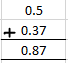So we get

0.5 + 0.37 = 0.87

(ii) 3.8 and 8.7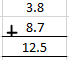So we get

3.8 + 8.7 = 12.5

(iii) 0.02, 0.008 and 0.309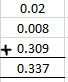So we get

0.02 + 0.008 + 0.309 = 0.337

(iv) 0.4136, 0.3195 and 0.52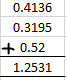So we get

0.4136 + 0.3195 + 0.52 = 1.2531

(v) 9.25, 3.4 and 6.666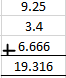So we get

9.25 + 3.4 + 6.666 = 19.316

(vi) 3.007, 0.587 and 18.341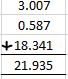So we get

3.007 + 0.587 + 18.341 = 21.935

(vii) 0.2, 0.02 and 2.0002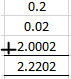So we get

0.2 + 0.02 + 2.0002 = 2.2202

(viii) 6.08, 60.8, 0.608 and 0.0608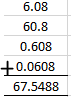So we get

6.08 + 60.8 + 0.608 + 0.0608 = 67.5488

(ix) 29.03, 0.0003, 0.3 and 7.2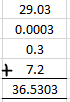So we get

29.03 + 0.0003 + 0.3 + 7.2 = 36.5303

(x) 3.4, 2.025, 9.36 and 3.6221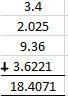So we get

3.4 + 2.025 + 9.36 + 3.6221 = 18.4071

2. Subtract the first number from the second:
(i) 5.4, 9.8
(ii) 0.16, 4.3
(iii) 0.82, 8.6
(v) 2.237, 9.425
(vi) 41 .03, 59.46
(vii) 3.92. 26.86
(viii) 4.73, 8.5
(ix) 12.63, 36.2
(x) 0.845, 3.71

Solution:

(i) 5.4, 9.8
It can be written as

9.8 – 5.4 = 4.4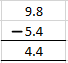(ii) 0.16, 4.3
It can be written as

4.3 – 0.16 = 4.14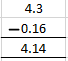(iii) 0.82, 8.6
It can be written as

8.6 – 0.82 = 7.78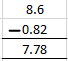(v) 2.237, 9.425
It can be written as

9.425 – 2.237 = 7.188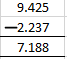(vi) 41 .03, 59.46
It can be written as

59.46 – 41.03 = 18.43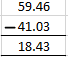(vii) 3.92, 26.86
It can be written as

26.86 – 3.92 = 22.94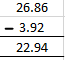(viii) 4.73, 8.5
It can be written as

8.5 – 4.73 = 3.77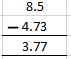(ix) 12.63, 36.2
It can be written as

36.2 – 12.63 = 23.57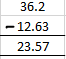(x) 0.845, 3.71

It can be written as

3.71 – 0.845 = 2.865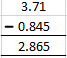3. Simplify:
(i) 28.796 -13.42 – 2.555
(ii) 93.354 – 62.82 – 13.045
(iii) 36 – 18.59 – 3.2
(iv) 86 + 16.95 – 3.0042
(v) 32.8 – 13 – 10.725 +3.517
(vi) 4000 – 30.51 – 753.101 – 69.43
(vii) 0.1835 + 163.2005 – 25.9 – 100
(viii) 38.00 – 30 + 200.200 – 0.230
(ix) 555.555 + 55.555 – 5.55 – 0.555

Solution:

(i) 28.796 -13.42 – 2.555
It can be written as

= 28.796 – (13.42 + 2.555)

On further calculation

= 28.796 – 15.975

= 12.821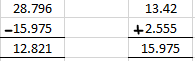(ii) 93.354 – 62.82 – 13.045
It can be written as

= 93.354 – (62.82 + 13.045)

On further calculation

= 93.354 – 75.865

= 17.489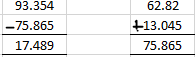(iii) 36 – 18.59 – 3.2
It can be written as

= 36 – (18.59 + 3.2)

On further calculation

= 36 – 21.79

= 14.21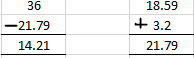(iv) 86 + 16.95 – 3.0042
It can be written as

= 102.95 – 3.0042

On further calculation

= 99.9458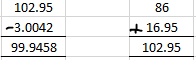(v) 32.8 – 13 – 10.725 +3.517
It can be written as

= (32.8 + 3.517) – (13 + 10.725)

On further calculation

= 36.317 – 23.725

= 12.592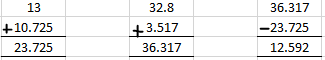(vi) 4000 – 30.51 – 753.101 – 69.43
It can be written as

= 4000 – (30.51 + 753.101 + 69.43)

On further calculation

= 4000 – 853.041

= 3146.959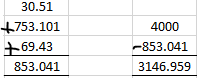(vii) 0.1835 + 163.2005 – 25.9 – 100
It can be written as

= (0.1835 + 163.2005) – (25.9 + 100)

On further calculation

= 163.2840 – 125.9

= 37.484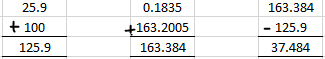(viii) 38.00 – 30 + 200.200 – 0.230
It can be written as

= (38.00 + 200.200) – (30 + 0.230)

On further calculation

= 238.200 – 30.230

= 207.970

= 207.97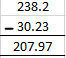(ix) 555.555 + 55.555 – 5.55 – 0.555

It can be written as

= (555.555 + 55.555) – (5.55 + 0.555)

On further calculation

= 611.110 – 6.105

= 605.005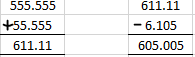4. Find the difference between 6.85 and 0.685.

Solution:

The difference between 6.85 and 0.685 = 6.85 – 0.685

= 6.165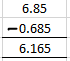5. Take out the sum of 19.38 and 56.025, then subtract it from 200.111.

Solution:

We know that the sum of 19.38 and 56.025 can be written as

19.38 + 56.025 = 75.405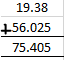We can write it as

Difference between 200.111 and 75.405

200.111 – 75.405 = 124.706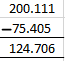6. Add 13.95 and 1.003, and from the result, subtract the sum of 2.794 and 6.2.

Solution:

We know that addition of 13.95 and 1.003 can be written as

13.95 + 1.003 = 14.953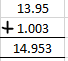Similarly the sum of 2.794 and 6.2 can be written as

2.794 + 6.2 = 8.994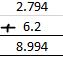Here the difference between 14.953 and 8.994

14.953 – 8.994 = 5.959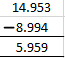7. What should be added to 39.587 to give 80.375?

Solution:

It is given that

Sum of two numbers = 80.375

One number = 39.587

So the other number = 80.375 – 39.587 = 40.788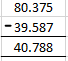8. What should be subtracted from 100 to give 19.29?

Solution:

It is given that

Sum of two numbers = 100

One number = 19.29

So the other number = 100 – 19.29 = 80.71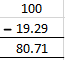9. What is the excess of 584.29 over 213.95?

Solution:

It is given that

Sum of two numbers = 584.29

One number = 213.95

So the other number = 584.29 – 213.95 = 370.34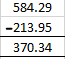10. Evaluate:

(i) (5.4 – 0.8) + (2.97 -1.462)

(ii) (6.25 + 0.36) – (17.2 – 8.97)

(iii) 9.004 + (3 -2.462)

(iv) 879.4 – (87.94 – 8 .794)

Solution:

(i) (5.4 – 0.8) + (2.97 -1.462)
It can be written as

= 4.6 + 1.508

On further calculation

= 6.108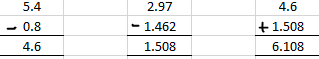(ii) (6.25 + 0.36) – (17.2 – 8.97)
It can be written as

= 6.61 – 8.23

On further calculation

= – 1.62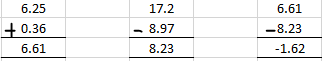(iii) 9.004 + (3 – 2.462)
It can be written as

= 9.004 + 0.538

On further calculation

= 9.542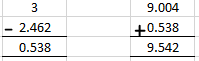(iv) 879.4 – (87.94 – 8 .794)

It can be written as

= 879.4 – 79.146

On further calculation

= 800.254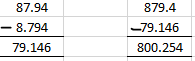11. What is the excess of 75 over 48.29?

Solution:

We know that the excess of 75 over 48.29 can be written as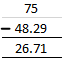Hence, the excess of 75 over 48.29 is 26.71.

12. If A = 237.98 and B = 83.47.

Find:

(i) A – B

(ii) B – A.

Solution:

(i) A – B

It is given that A = 237.98 and B = 83.47

Substituting the values

A – B = 237.98 – 83.47

A – B = 154.51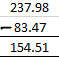(ii) B – A

It is given that A = 237.98 and B = 83.47

Substituting the values

B – A = 83.47 – 237.98

B – A = – 154.51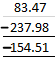13. The cost of one kg of sugar increases from 28.47 to 32.65. Find the increase in cost.

Solution:

Cost of sugar = 28.47

Cost of sugar is raised = 32.65

Increase in the cost of sugar = 32.65 – 28.47 = 4.18